# IP-based Geolocation in ClickHouseZach Naimon
May 23, 2023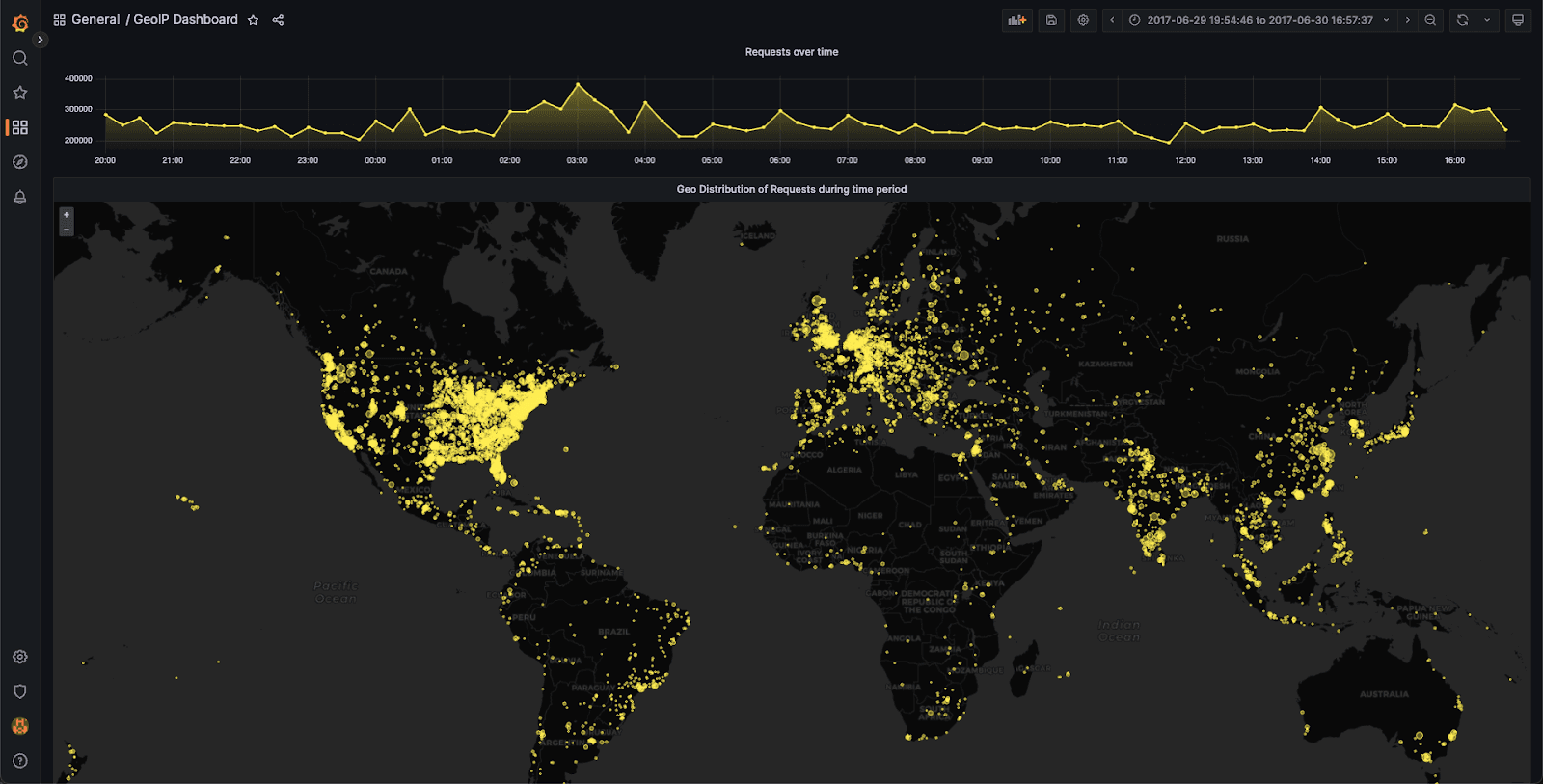## Introduction

ClickHouse is an open-source column-oriented database management system that was originally developed to power web analytics services such as Google Analytics. Many of ClickHouse's original features were developed to help users make sense of their web analytics data in real-time. One such feature is its powerful IP-based lookup feature.

In this blog post, we will explore how to use ClickHouse's `ip_trie` structured dictionary to perform IP-based geolocation. We will start by importing an open-source GeoIP dataset into ClickHouse and using bit functions to transform the data into a usable format. We will then create an `ip_trie` dictionary from our dataset and use the `dictGet()` function to determine the approximate location of a given IP address.

Finally, we will explore how to use geo hashing to aggregate geospatial data and create rich visualizations in tools like Grafana. With these tools at our disposal, we can gain valuable insights into the geographic distribution of our users and better understand how they interact with our websites and applications. So let's get started and learn how to leverage the power of ClickHouse for IP-based geolocation!

While we use ClickHouse Cloud for our examples, they should be reproducible on a self-managed cluster. Alternatively, start a ClickHouse Cloud trial today, receive \$300 of free credit, leave the infrastructure to us and get querying!

## Importing and Transforming GeoIP Data in ClickHouse

For this blog post, we’ll use the publicly available DB-IP city-level dataset provided by DB-IP.com under the terms of the CC BY 4.0 license.

### Previewing the GeoIP Data using url()

From the readme, we can see that the data is structured as follows:

``````| ip_range_start | ip_range_end | country_code | state1 | state2 | city | postcode | latitude | longitude | timezone |
``````

Given this structure, let’s start by taking a peek at the data using the url() table function using the SQL console in ClickHouse Cloud:

``````select
*
from
url(
'https://raw.githubusercontent.com/sapics/ip-location-db/master/dbip-city/dbip-city-ipv4.csv.gz',
'CSV',
'
ip_range_start IPv4,
ip_range_end IPv4,
country_code Nullable(String),
state1 Nullable(String),
state2 Nullable(String),
city Nullable(String),
postcode Nullable(String),
latitude Float64,
longitude Float64,
timezone Nullable(String)
'
)
limit
20;
``````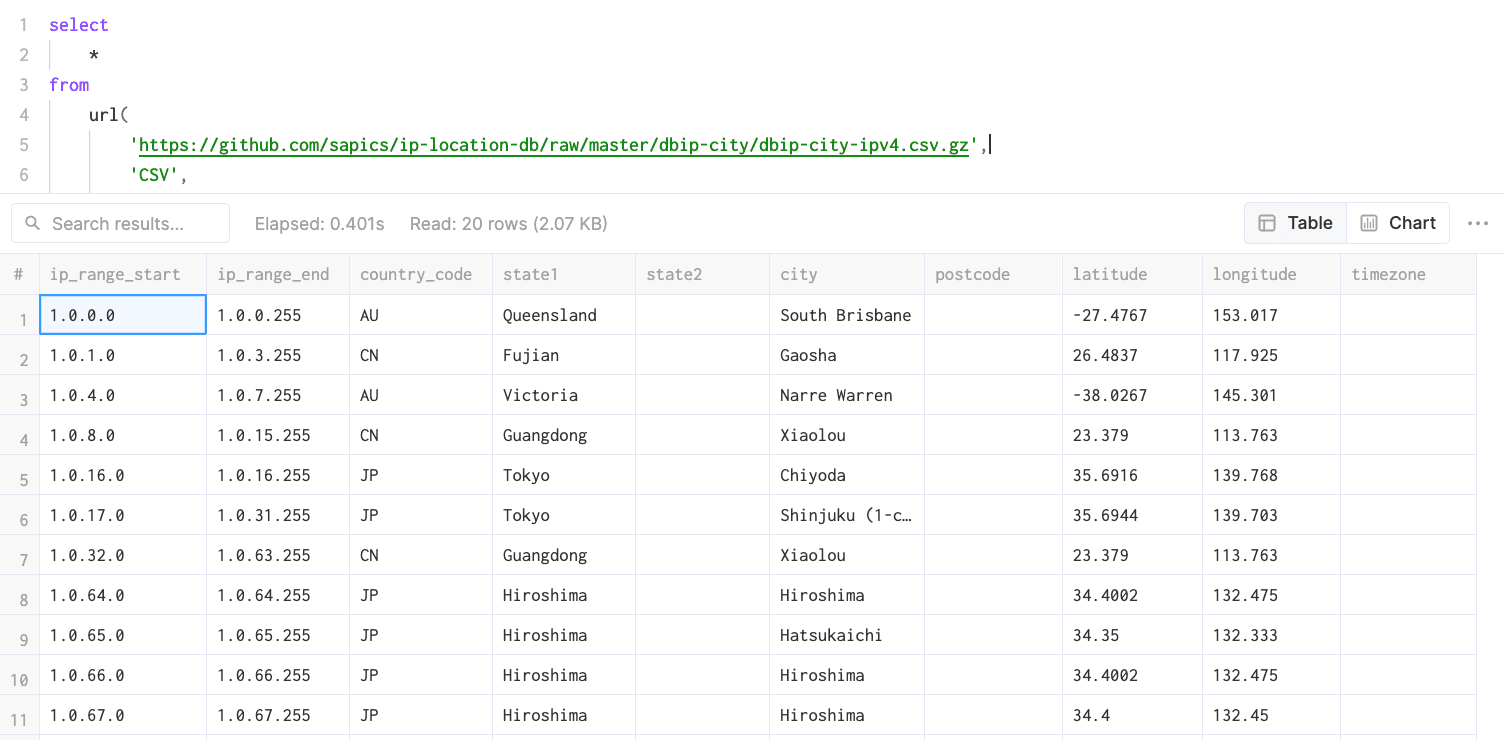To make our lives easier, let’s use the `URL()` table engine to create a ClickHouse table object with our field names:

``````create table geoip_url(
ip_range_start IPv4,
ip_range_end IPv4,
country_code Nullable(String),
state1 Nullable(String),
state2 Nullable(String),
city Nullable(String),
postcode Nullable(String),
latitude Float64,
longitude Float64,
timezone Nullable(String)
) engine=URL('https://raw.githubusercontent.com/sapics/ip-location-db/master/dbip-city/dbip-city-ipv4.csv.gz', 'CSV');
``````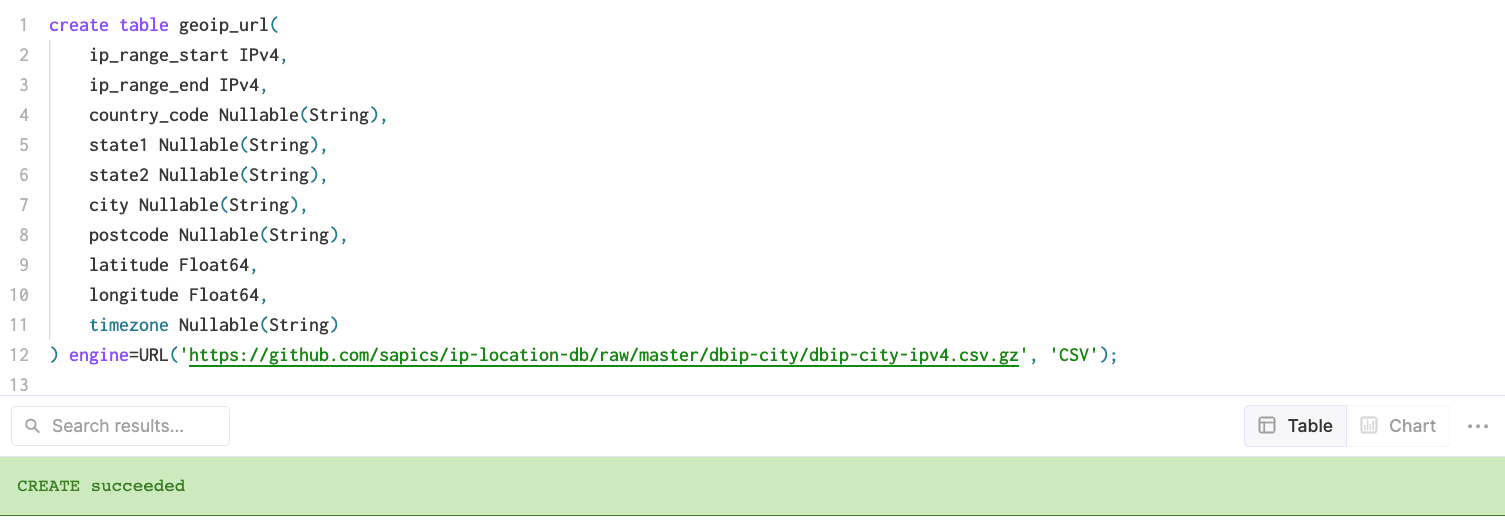Let’s also check how many records are contained in the dataset:

``````select count() from geoip_url;
``````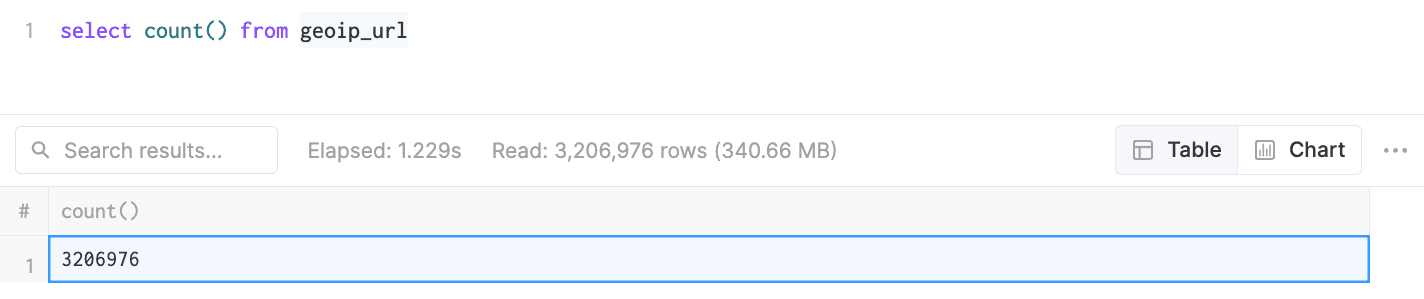### Using Bit Functions to Convert IP Ranges to CIDR Notation

Because our `ip_trie` dictionary requires IP address ranges to be expressed in CIDR notation, we’ll need to transform `ip_range_start` and `ip_range_end`. For reference, here’s a brief description of CIDR notation from Wikipedia:

These groups, commonly called CIDR blocks, share an initial sequence of bits in the binary representation of their IP addresses. IPv4 CIDR blocks are identified using a syntax similar to that of IPv4 addresses: a dotted-decimal address, followed by a slash, then a number from 0 to 32, i.e., a.b.c.d/n. The dotted decimal portion is the IPv4 address. The number following the slash is the prefix length, the number of shared initial bits, counting from the most-significant bit of the address. When emphasizing only the size of a network, the address portion of the notation is usually omitted. Thus, a /20 block is a CIDR block with an unspecified 20-bit prefix.

An IP address is part of a CIDR block and is said to match the CIDR prefix if the initial n bits of the address and the CIDR prefix are the same. An IPv4 address is 32 bits so an n-bit CIDR prefix leaves 32 − n bits unmatched, meaning that 232−n IPv4 addresses match a given n-bit CIDR prefix.

An IP address is part of a CIDR block and is said to match the CIDR prefix if the initial n bits of the address and the CIDR prefix are the same. An IPv4 address is 32 bits so an n-bit CIDR prefix leaves 32 − n bits unmatched, meaning that 232−n IPv4 addresses match a given n-bit CIDR prefix.

In order to generate a CIDR expression for our IP ranges, we’ll need to compare each range to determine unmatched bits in each address and then determine the smallest possible CIDR range that encompasses the whole range. Here’s a diagram to demonstrate: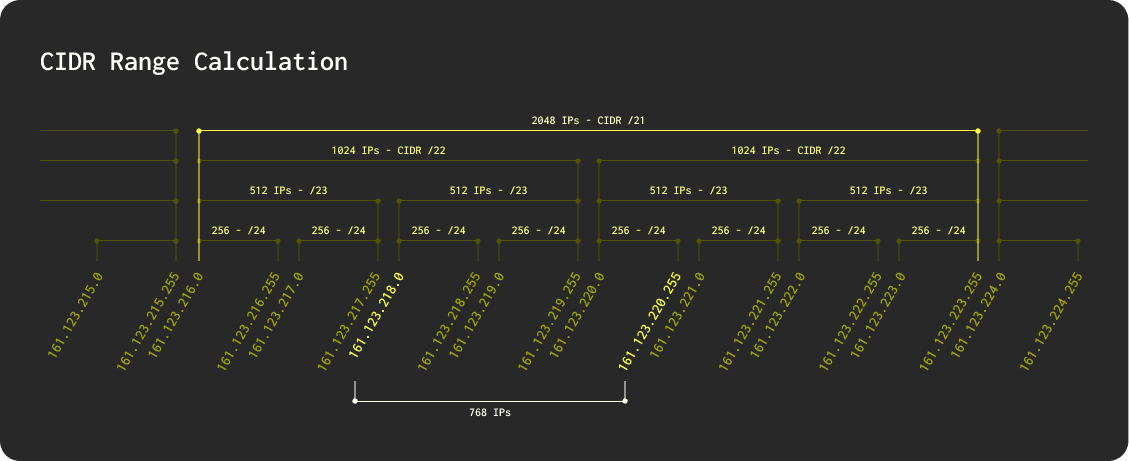In this diagram, we can see that our example IP address range (`161.123.218.0``161.123.220.255`) contains 768 possible addresses, spanning three /24 CIDR blocks, two /23 blocks, and two /22 blocks. As a result, the smallest possible CIDR block that contains the range is a /21 block (containing 2048 addresses). Amazingly, this entire calculation can be accomplished using a handful of bitwise and mathematical functions supported by ClickHouse.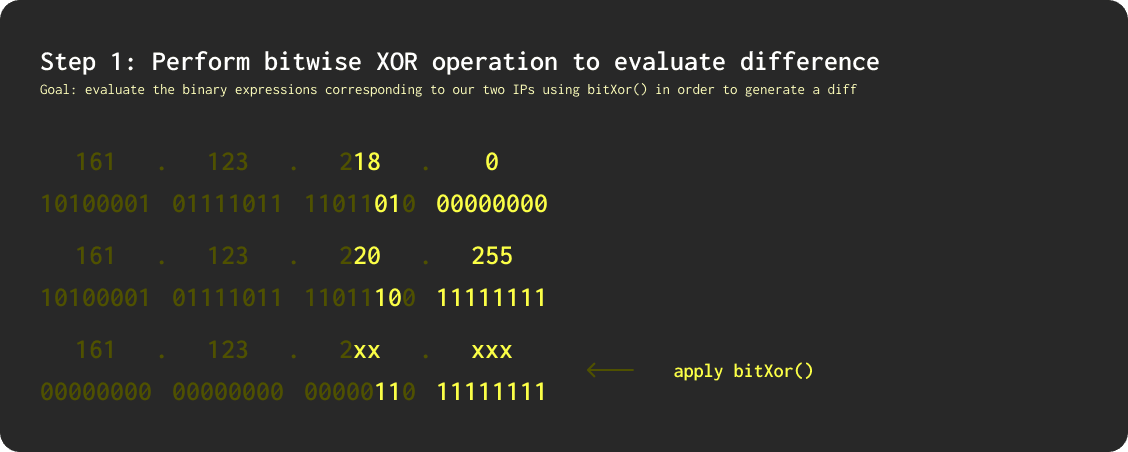To begin, we’ll use the `bitXor()` function in ClickHouse, which performs a bitwise XOR operation between two numbers and returns the result of the XOR operation. The XOR operation compares each bit of the two numbers, outputting 1 if the two digits are different and 0 if they are the same. For example, the expression `bitXor(5,3)` would evaluate to `6` since 5 is represented in binary as `101` and 3 is represented as `011`. Comparing each bit produces `110` which is equivalent to `6` in decimal. Let’s see what happens when we use cast our IPs as Integers and apply the `bitXor()` function—note that ClickHouse automatically converts bitXor() outputs into decimal form, so we’ve added an additional column to demonstrate the underlying binary expression:

``````select
ip_range_start,
ip_range_end,
bitXor(ip_range_start, ip_range_end) as xor,
bin(xor) as xor_binary
from
geoip_url
limit
20;
``````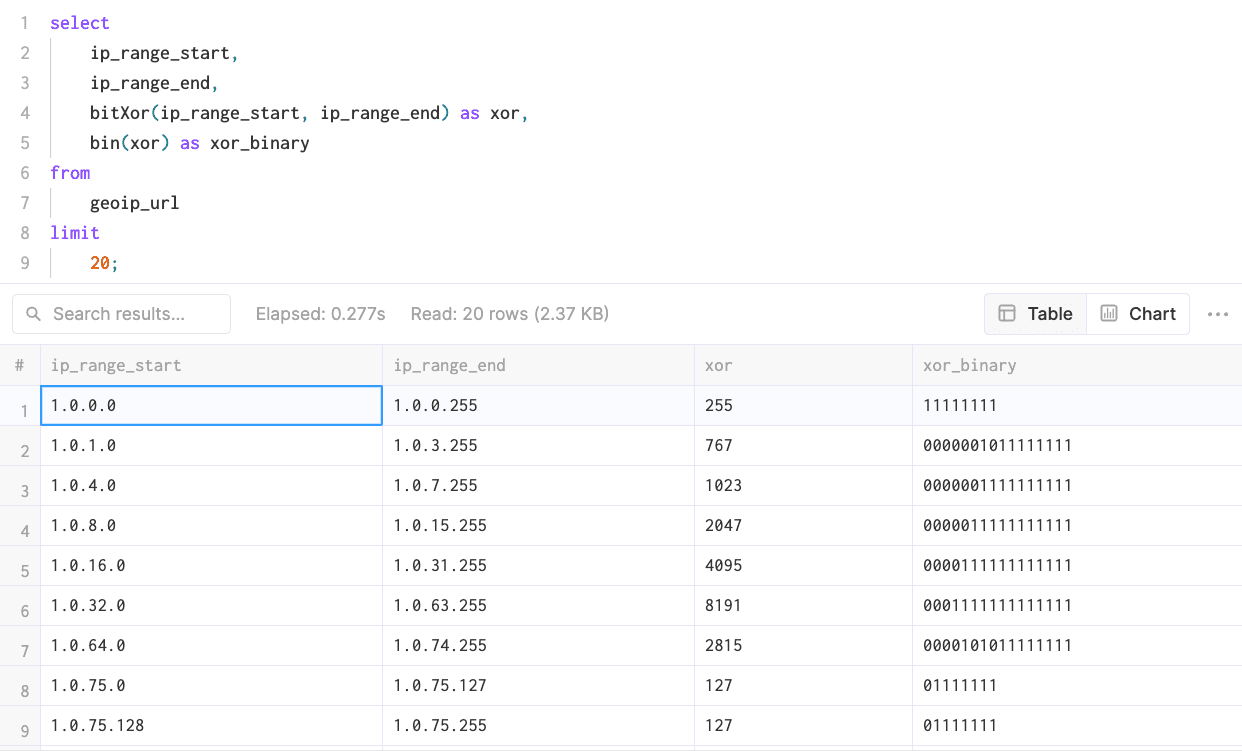From here, we can see that `bitXor()` will return the number of addresses in our range (for example 255 between `1.0.0.0` and `1.0.0.255`).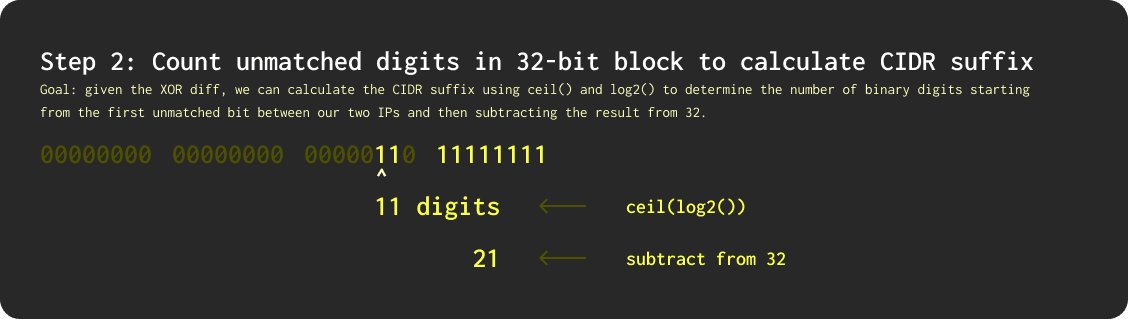Next, we’ll use `log2()` and `ceil()` to count the number of binary digits beginning with the first unmatched bit. We’ll also need to handle scenarios where the start and end of our IP range are equal (thereby yielding a xor output of 0) because `log2(0)` is undefined:

``````select
ip_range_start,
ip_range_end,
bitXor(ip_range_start, ip_range_end) as xor,
bin(xor) as xor_binary,
if(xor != 0, ceil(log2(xor)), 0) as unmatched,
32-unmatched as cidr_suffix
from
geoip_url
limit
20;
``````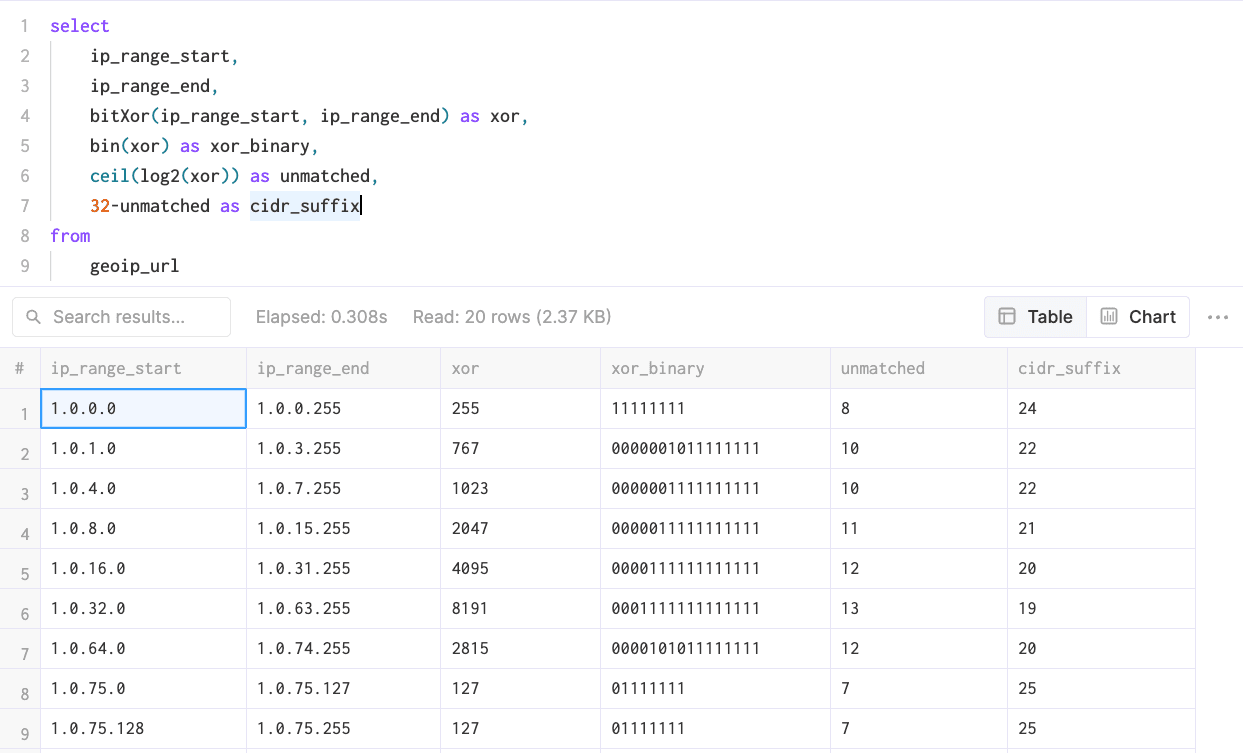In the results above, we can see that the `unmatched` field is effectively calculating the position of the first `1` bit from our `bitXor()` output. Subtracting this value from 32 yields our CIDR block size (suffix).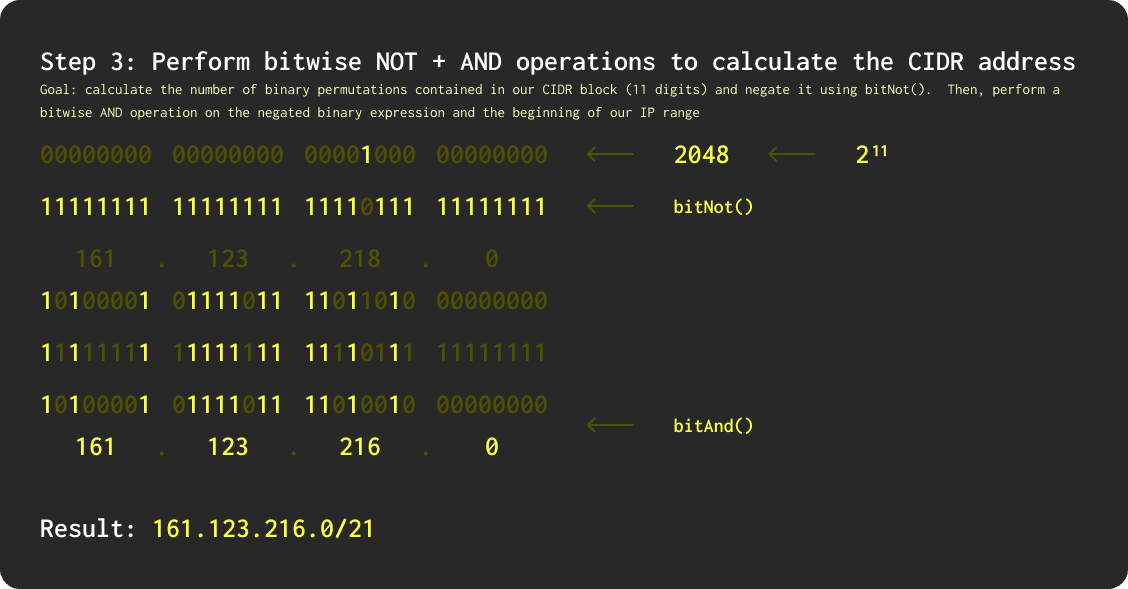Next, we’ll determine the address at which the CIDR block begins, as this will often differ from the address at the beginning of our range, especially for larger blocks. To do this, we’ll need to perform a bitwise NOT operation `bitNot()` on the total number of addresses contained in our CIDR block, which can be expressed as `pow(2, n) - 1` where `n` is equal to the value of our `unmatched` field above. Then, we’ll compare the result with the beginning of our IP range using a bitwise AND operation `bitAnd()` to determine which bits in both expressions are equal to 1. The result can then be converted back into an IPv4 address by first casting it to `UInt64` and then applying the `toIPv4()` function:

``````select
ip_range_start,
ip_range_end,
bitXor(ip_range_start, ip_range_end) as xor,
bin(xor) as xor_binary,
if(xor != 0, ceil(log2(xor)), 0) as unmatched,
32 - unmatched as cidr_suffix,
toIPv4(bitAnd(bitNot(pow(2, unmatched) - 1), ip_range_start)::UInt64) as cidr_address
from
geoip_url
limit
20;
``````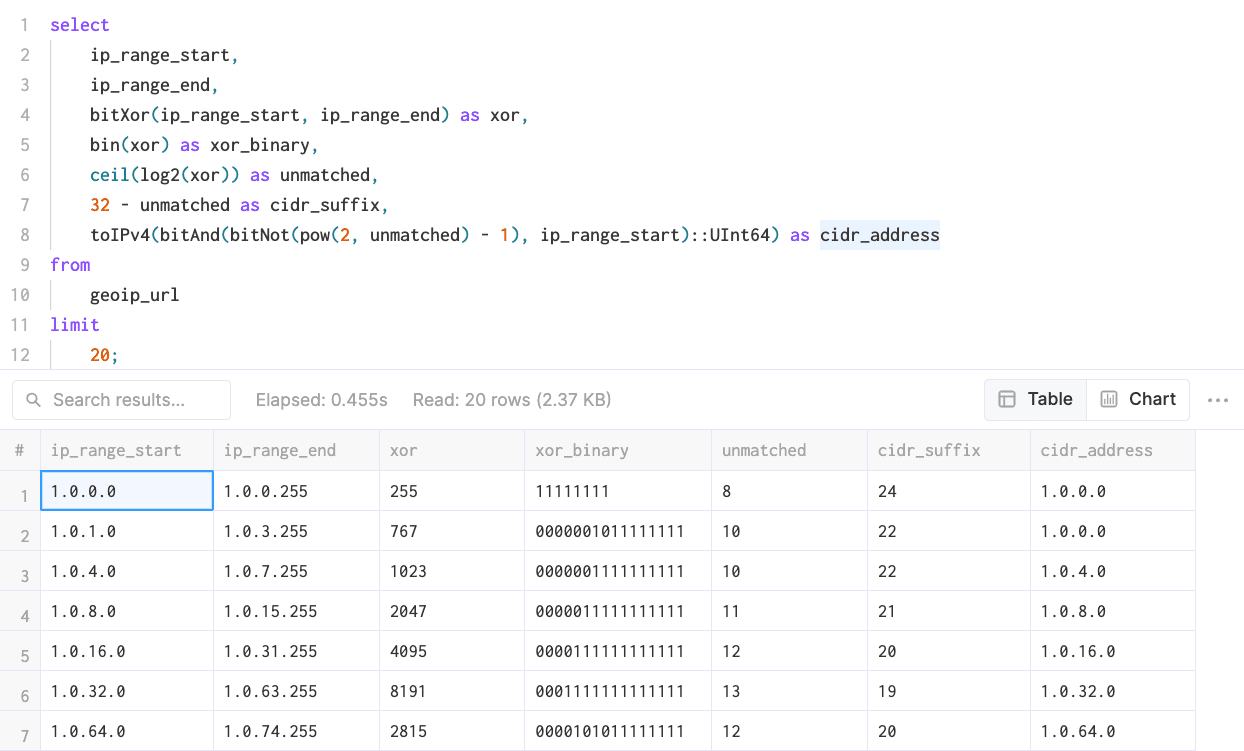Finally, we can concatenate our CIDR address and suffix into a single string. To make this clean, we’ll also move all of our intermediary expressions into a `WITH` clause.

``````with
bitXor(ip_range_start, ip_range_end) as xor,
if(xor != 0, ceil(log2(xor)), 0) as unmatched,
32 - unmatched as cidr_suffix,
toIPv4(bitAnd(bitNot(pow(2, unmatched) - 1), ip_range_start)::UInt64) as cidr_address
select
ip_range_start,
ip_range_end,
from
geoip_url
limit
20;
``````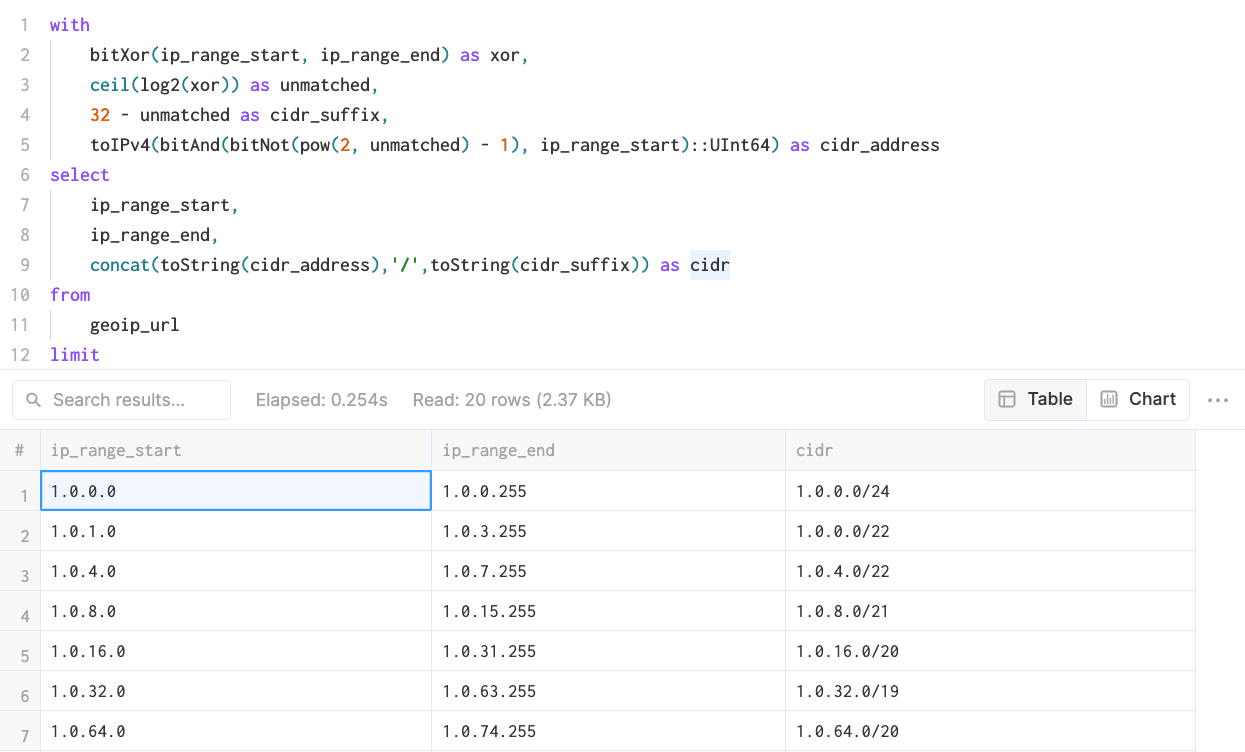### Importing the transformed GeoIP Data

For our purposes, we’ll only need the IP range, country code and coordinates, so let’s create a new table and insert our GeoIP data:

``````create table geoip (
cidr String,
latitude Float64,
longitude Float64,
country_code String
)
engine = MergeTree()
order by cidr;

insert into
geoip
with
bitXor(ip_range_start, ip_range_end) as xor,
if(xor != 0, ceil(log2(xor)), 0) as unmatched,
32 - unmatched as cidr_suffix,
toIPv4(bitAnd(bitNot(pow(2, unmatched) - 1), ip_range_start)::UInt64) as cidr_address
select
latitude,
longitude,
country_code
from
geoip_url
``````

## Creating an `ip_trie` Dictionary for GeoIP Data

In order to perform low-latency IP lookups in ClickHouse, we’ll leverage dictionaries to store `key -> attributes` mapping for our GeoIP data in-memory. ClickHouse provides an `ip_trie` dictionary structure to map our network prefixes (CIDR blocks) to coordinates and country codes:

``````create dictionary ip_trie (
cidr String,
latitude Float64,
longitude Float64,
country_code String
)
primary key cidr
source(clickhouse(table ‘geoip’))
layout(ip_trie)
``````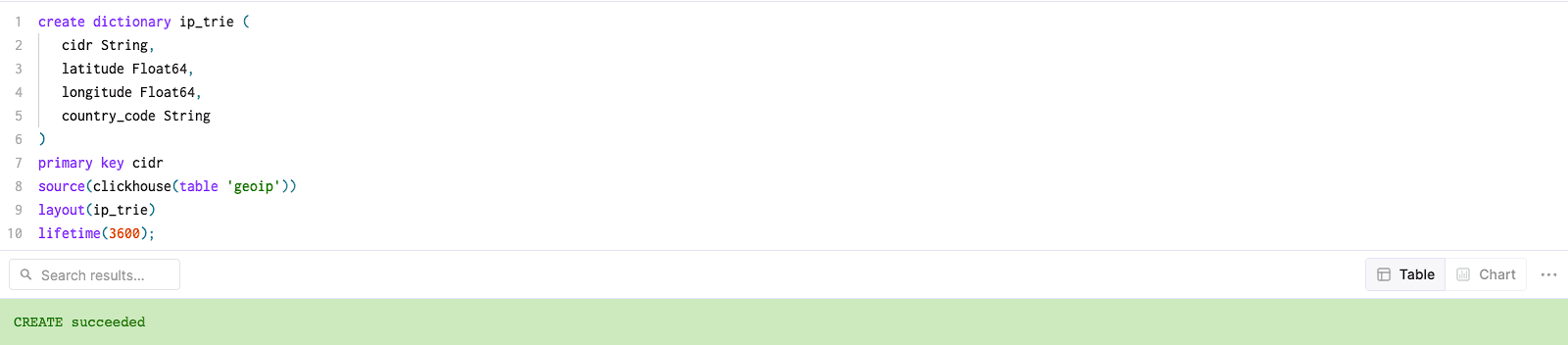Just to confirm that everything has been correctly loaded into the dictionary, let’s take a quick peek:

``````select * from ip_trie limit 20;
``````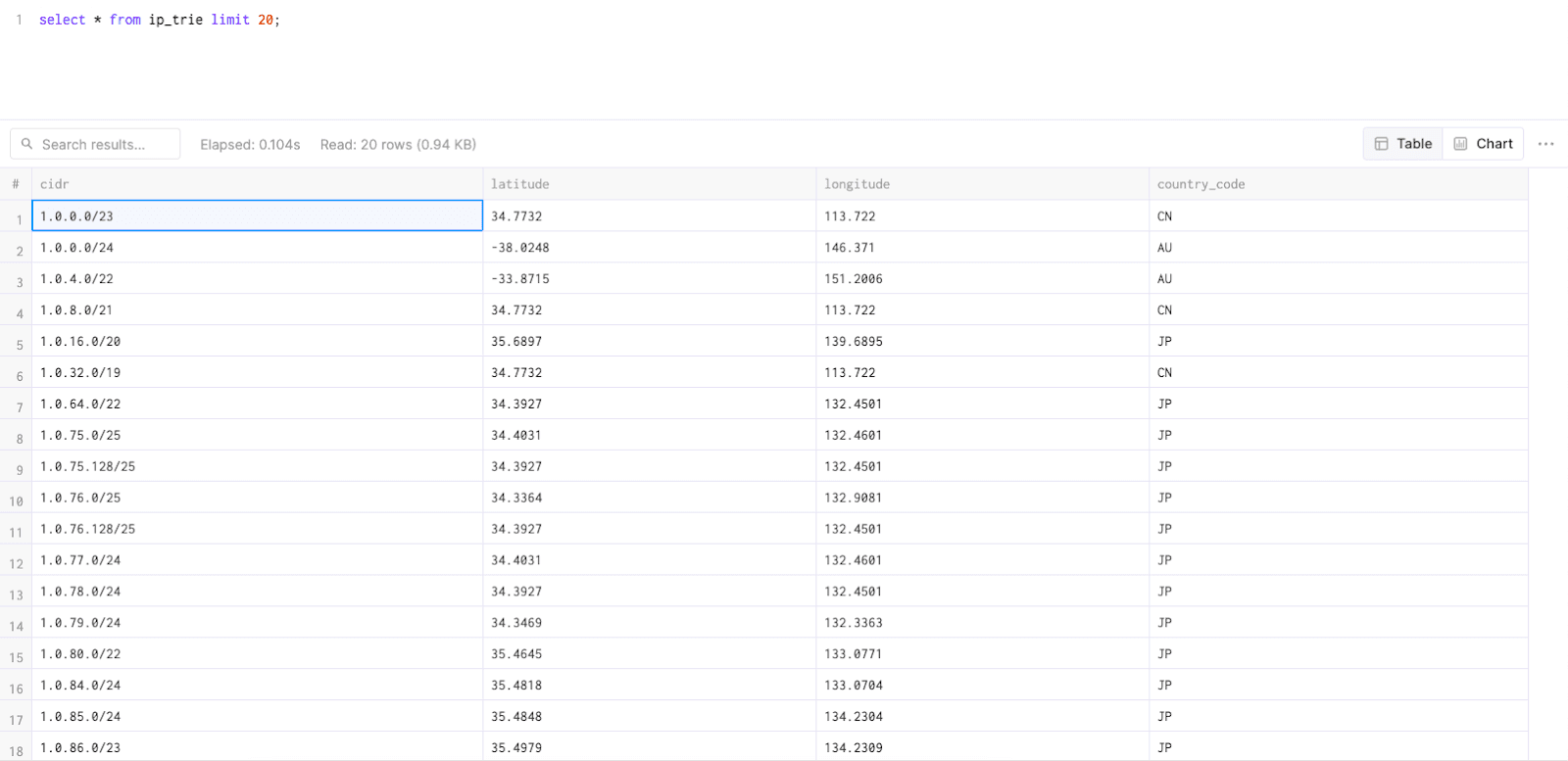Dictionaries in ClickHouse are periodically refreshed based on the underlying table data and the lifetime clause used above. In order to update our GeoIP dictionary to reflect the latest changes in the DB-IP dataset, we’ll just need to reinsert data from the `geoip_url` remote table to our `geoip` table with transformations applied.

## Performing IP Lookups from an `ip_trie` Dictionary

Now that we have GeoIP data loaded into our `ip_trie` dictionary (conveniently also named `ip_trie`), we can start using it for IP geolocation. This can be accomplished using the `dictGet()` function as follows:

``````dictGet(
‘<dictionary name>’,
(‘field1’,’field2’, .. .’fieldN’),
tuple(<ip address in type IPV4 or UInt>)
)
``````

To begin, let’s try looking up a single IP: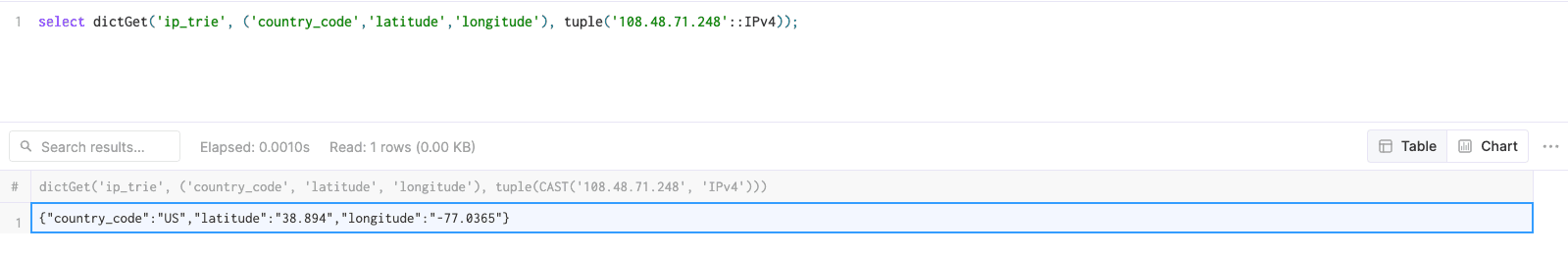As we can see, `dictGet()` is returning a tuple of all specified fields contained in our dictionary for this IP. Let’s now apply this lookup on a table containing many IP addresses. For this demo, we’ll use an excerpt from the ‘EDGAR Log File Data Sets’ published by the SEC.

``````select
datetime,
ip,
accession,
extention,
dictGet(
'ip_trie',
('country_code', 'latitude', 'longitude'),
tuple(ip)
) as geo_info
from
edgar
where
geo_info.1 != ''
limit
1000;
``````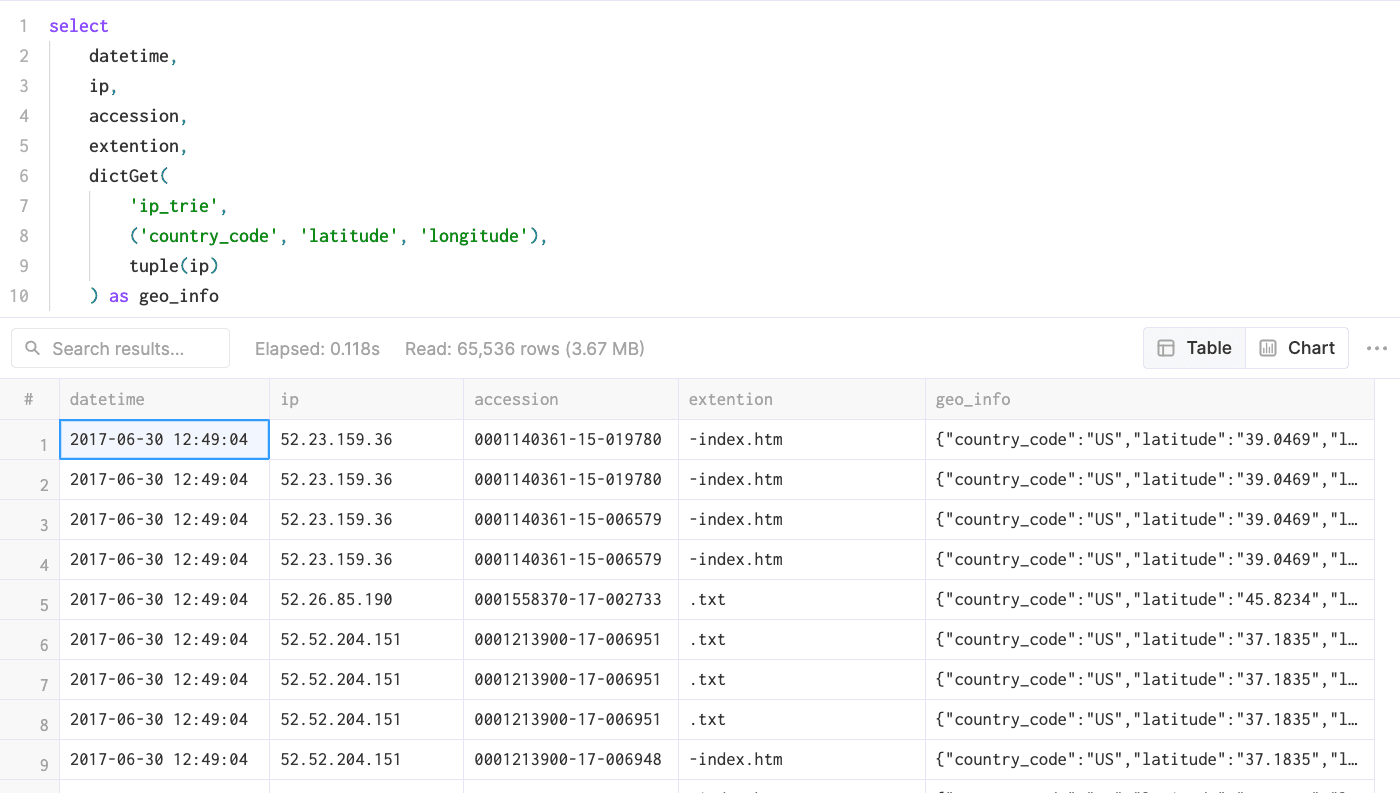Inevitably, this data is much better interpreted using aggregations, so let’s write a query to determine the country-level breakdown of access log events:

``````select
dictGet(
'ip_trie',
('country_code', 'latitude', 'longitude'),
tuple(ip)
).1 as country,
from edgar
where country != ''
group by country
order by count() desc
``````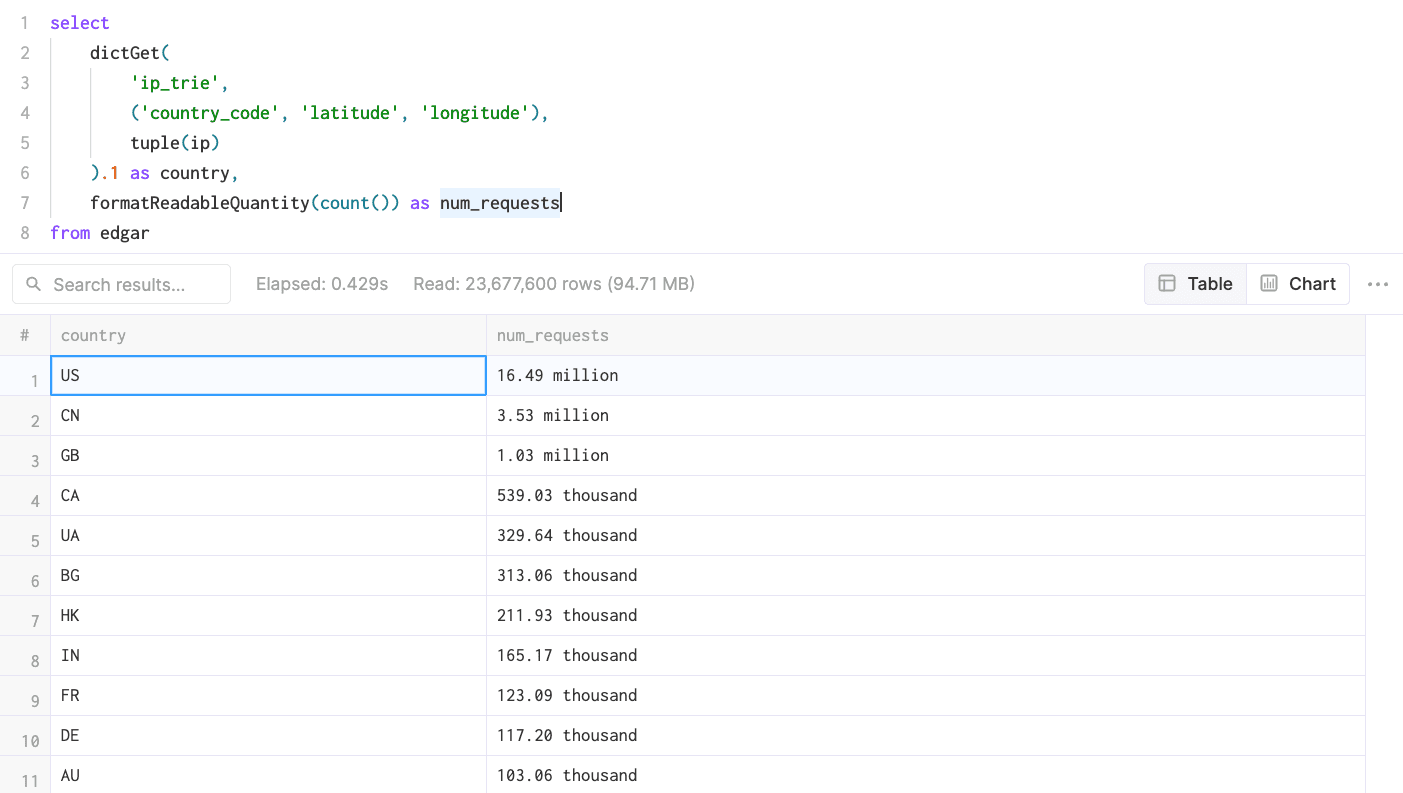## Aggregating Geospatial Data for Visualizations

Tools like Grafana and Metabase can help us generate geospatial visualizations from our latitude/longitude coordinates, but there is one dilemma: plotting tens of millions of points on the map is prohibitively expensive. Thus, we need to pre-aggregate our geodata result set before rendering a visualization. To do this, we’ll create geohashes from our coordinates using `geohashEncode()` with a low value for the `precision` parameter, aggregate over this hash, and use the result of the `count()` function with logarithmic normalization `log10()` to show density while making sure our heat scores scale nicely:

``````with coords as (
select
dictGet(
'ip_trie',
('latitude', 'longitude'),
tuple(ip)
) as coords,
coords.1 as latitude,
coords.2 as longitude,
geohashEncode(longitude,latitude,4) as hash
from
edgar
where
longitude != 0
and latitude != 0
)
select
hash,
count() as heat,
from
coords
group by
hash
``````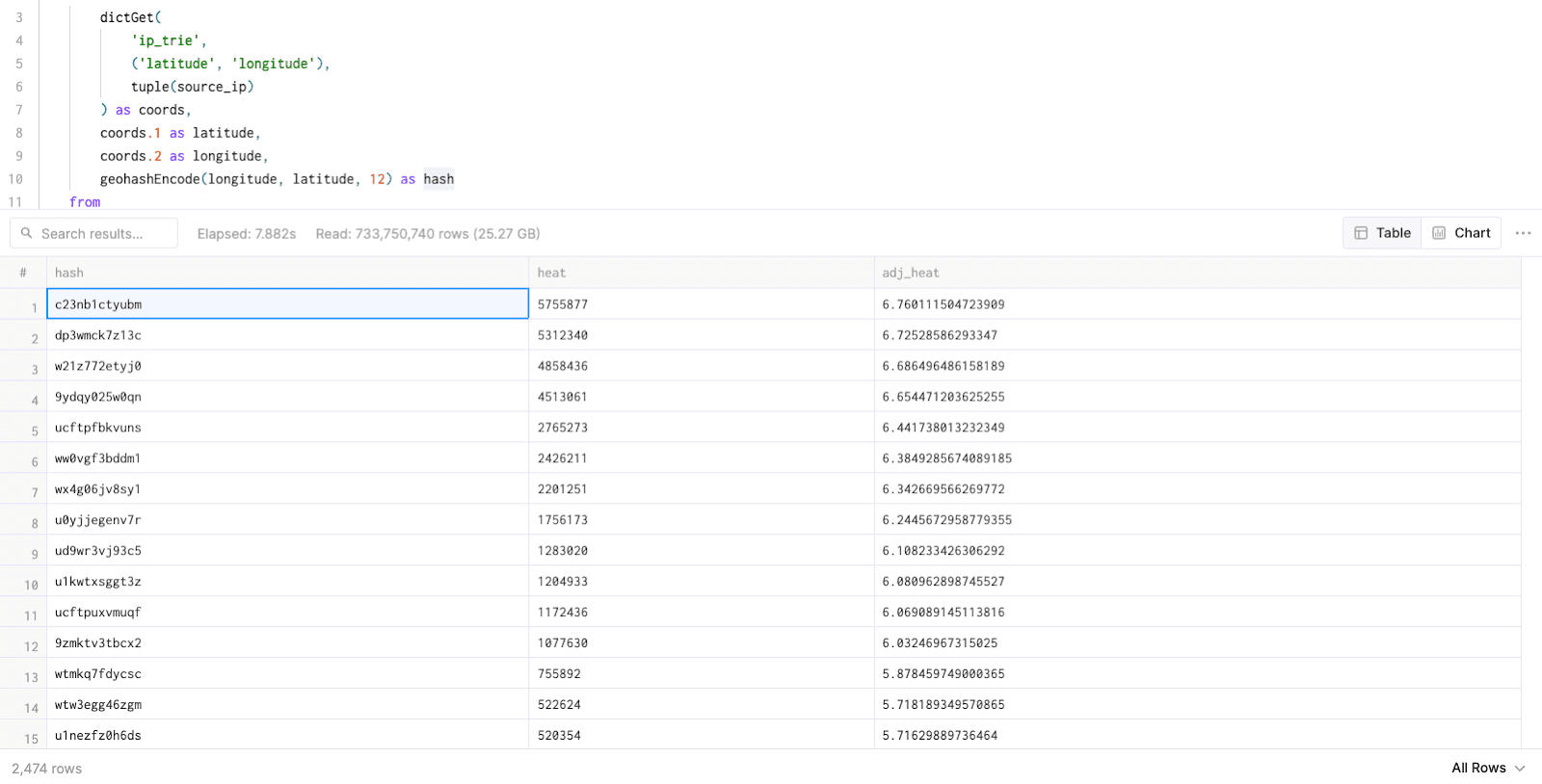Using geo-hashing, we’ve now rolled up 20M+ records into ~6300 results. Loading this query into Grafana to power a `Geo Map` chart panel will produce a rich visualization:NB: we can also add a `\$__timeFilter()` macro to the query in Grafana to scope our results to a specific time filter. You can read more about leveraging Grafana with ClickHouse in this blog. Similar results can be achieved in Metabase as well.